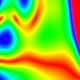• Metals

What I want to do is work out what is the total weight of each element in the lithosphere.

I’ve a list of elemental abundance in said lithosphere here.

I also know that the weight of the lithosphere is 1.5 x 10 to the 23 kg.

And I want the answer in tonnes.

This is entirely obvious therefore: multiply the abundance in parts per million (as measured by weight, of course) by the weight of the lithosphere. And do that for each element.

But I’m getting entirely lost in a welter of zeros all floating about. Is 1.5 x 10 to the 23 kg actually 1.5 x 10 to the 20, or to the 19 tonnes? Is one part per million 10 to the minus 6 tonnes or minus 7?

I tried cranking through this and it tells me that rhenium (0.0004 ppm) is actually 6 billion tonnes. And that just doesn’t seem right.

I’m sure this is something that is relatively trivial to do in a spreadsheet but I don’t know how to use one of those.

All I want is element by ppm in the lithosphere times weight of the lithosphere. With the answer in metric tonnes.

Err?

13 thoughts on “I had forgotten how appallingly bad at numbers I am”

1.Convention 1 E+x = 1 x 10 to the x

1 tonne = 1 E+3 kg (1,000)
Earth’s crust = 1.5 E +23 kg = 1.5 E +20 tonnes
1 ppm = 1 E-6
1ppm of the Earths crust = 1.5 E+14 tonnes

so to get the number of tonnes of an element, just multiply the ppm by 1.5 E+14

e.g. rhenium 4 E-4 = 6 E+10 tonnes = 60 thousand million tonnes

2.1.5E23 kg = 1.5E20 tonnes
1 ppm = 1E-6
0.0004 ppm = 4E-10
Abundance in lithosphere = 1.5E20 tonnes * 4E-10 = 6E10 tonnes = 60 billion tonnes.

The lithosphere is about 10 times bigger than the crust, of course.

3.By the way, a more accurate figure for the weight of the Earth’s crust according to here:

is 2.77 E +22. Change cell B1 in that spreadsheet to use a different value for the crust weight, the rest will update.

4.weight^W mass

/pendant

5.Oh, don’t be a spoilsport, ukl: there’s something charmingly old-fashioned in chattering about “weight`’.

6.Tim, 10 to the N means a 1 with N zeros after it. 10 to the 1 is 10. 10 to the 6 is 1 million. Etc.

7.So, 10 to the 0 is 1. 10 to the -1 is 0.1, 10 to the -2 is .01, etc.

8.You could use Wolfram Alpha. It’s what it’s for.

If the lithosphere is 2.77 × 10^22 kg (about 0.5% of Earth’s mass) then 0.0004 ppm gives us about 11 billion tons. Rhenium’s dense (21020 kg/m^3) so that’s only 530 million cubic meters in its elemental form or a cube about 800m on a side. A rule of thumb is that each part per million is about 28 trillion tonnes.

9.do you want fries with that?

10.Fairly simple, it seems. Calculate the total weight of the lithosphere, multiply by the 0.111 (or whatever) of the element you’re looking for. Proportions will add up to 100%.

But we don’t know beyond a few miles down, do we? It might be like the bits we know, but it might not.

Is Timmy planning to divert an asteroid to strike the Himalayas or the Antarctic in order to overturn the earth’s crust and get some more of his beloved Scandium out? I think we should be told.

11.First time I skied the Vallee Blanche there was a big rock on the glacier just below the “salle a manger”. I assumed it would be subducted into the bottom of the galcier, as rocks are denser than ice,

Years later, that rock is still on the surface. It’s a lump. You can see the same effect by shaking coffee grounds.

The lithosphere is a bit like a glacier, but on billion rather than thousand year time scale. Lumpy stuff will stay close to the surface, small stuff will descend, larger molecules will resurface faster than small ones, etc.

If you’ve got a ground penetrating radar that can see through a few miles, then Tim Newman wants to hear from you. Until then, the composition of the lithosphere is going to remain speculative.

12.But don’t get carried away! You would have to process the entire planet to get at it, and the average concentration is so low it is below normal measurement threshold.
I would guess that some of it is concentrated so the rest is at even lower ppm.
Seawater is something that you could actually obtain to process even if it would be uneconomic: 7ng/kg rhenium content or around 10million tonnes.